# C1=0.1μFandC2=0.1μF solve for V1,V2,V3,V4 V1 R1 1.IK V2 R3 1.7K V3 RS 3.0K R6 5.7K VSI...

C1=0.1μFandC2=0.1μF

solve for V1,V2,V3,V4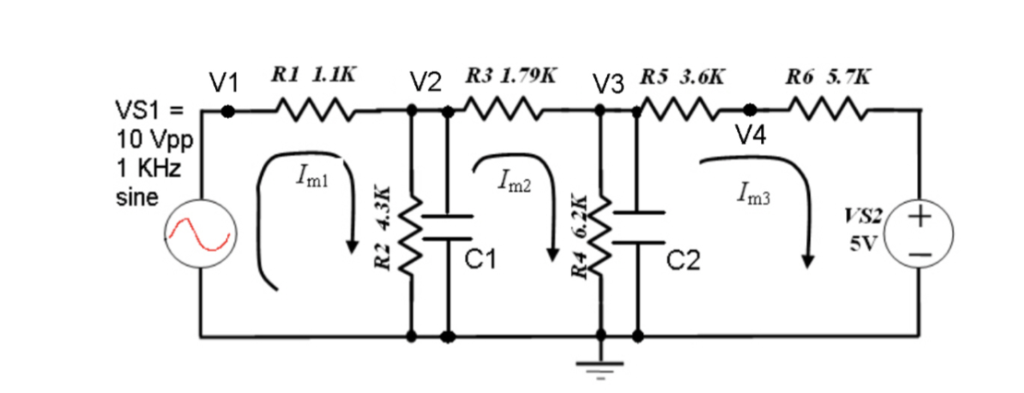V1 R1 1.IK V2 R3 1.7K V3 RS 3.0K R6 5.7K VSI 10 Vpp 1 KHz sine V4. Im1 Im2 Im3 5V C2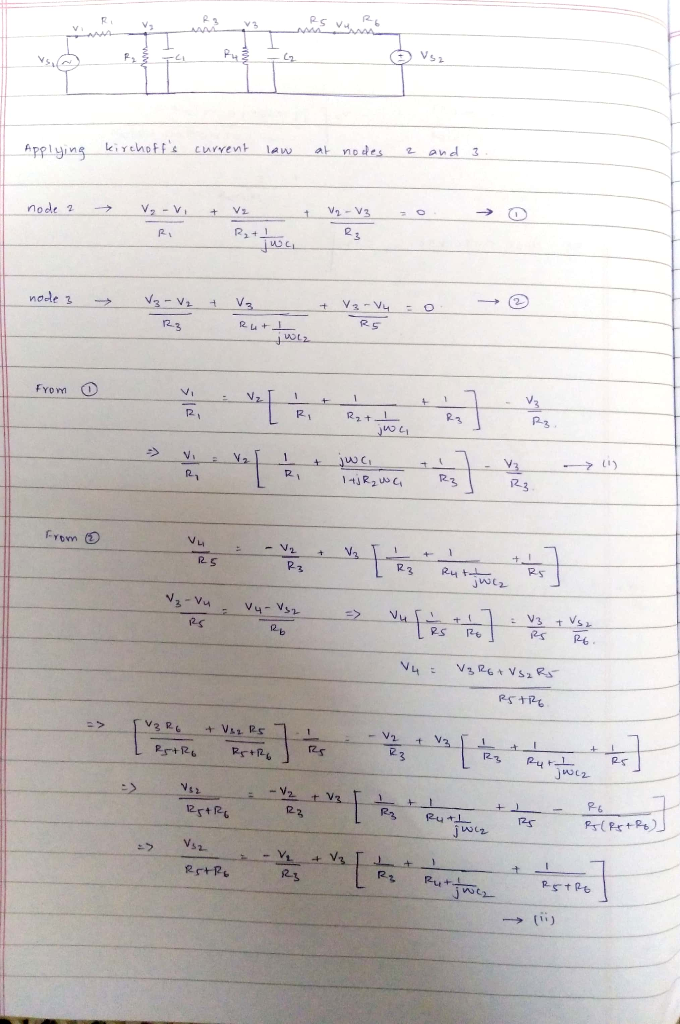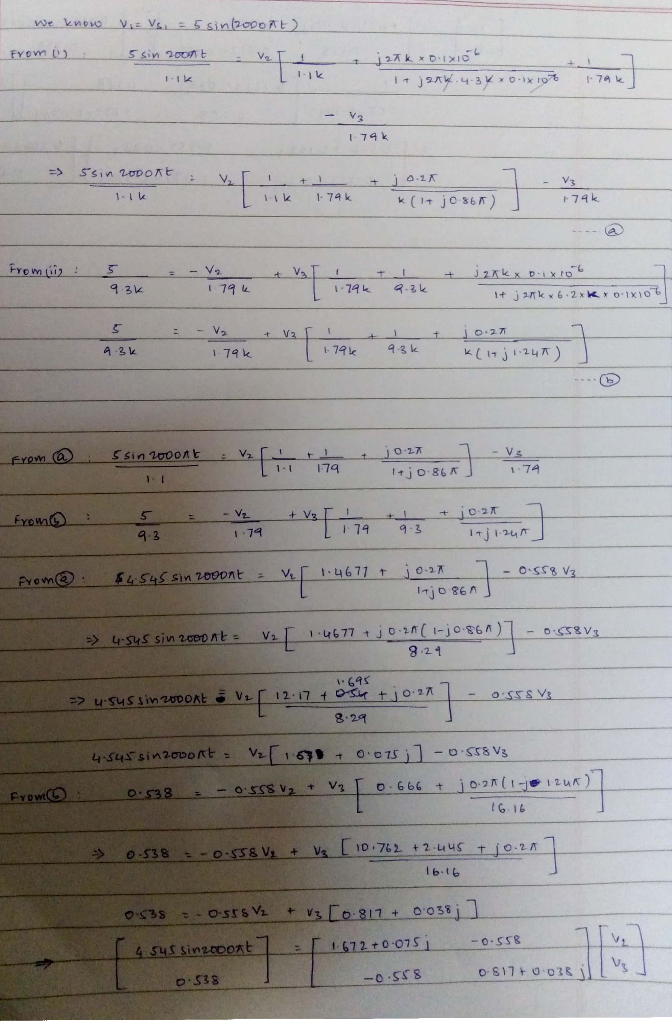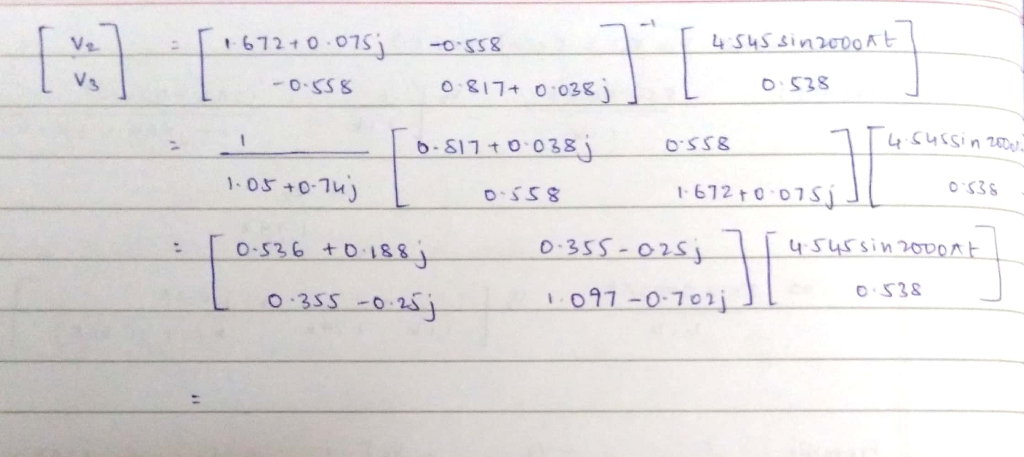#### Earn Coin

Coins can be redeemed for fabulous gifts.

Similar Homework Help Questions
• ### Let H = Span{V1, V2} and K = Span{V3,V4}, where V1, V2, V3, and V4 are...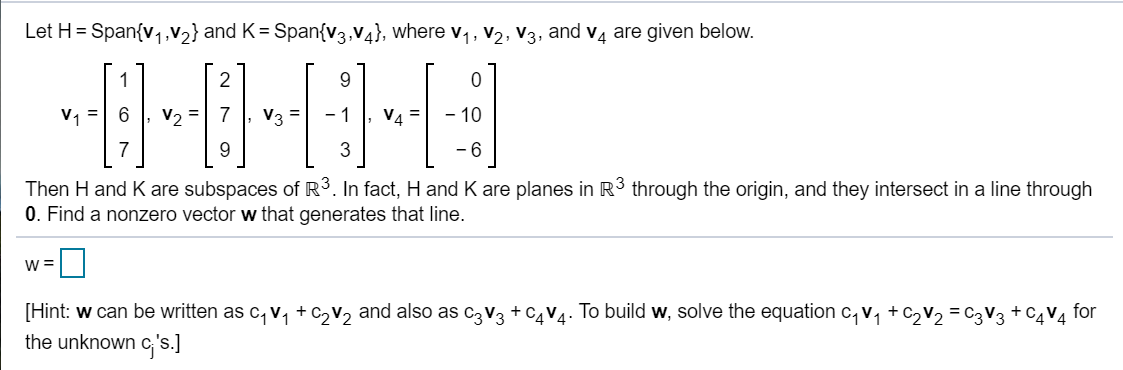Let H = Span{V1, V2} and K = Span{V3,V4}, where V1, V2, V3, and V4 are given below. 1 V1 V2 V4 - 10 7 9 3 -6 Then Hand K are subspaces of R3. In fact, H and K are planes in R3 through the origin, and they intersect in a line through 0. Find a nonzero vector w that generates that line. W= [Hint: w can be written as C1 V2 + c2V2 and also as c3 V3...

• ### Let H = Span{V1, V2} and K = Span{V3,V4}, where V1, V2, V3, and V4 are...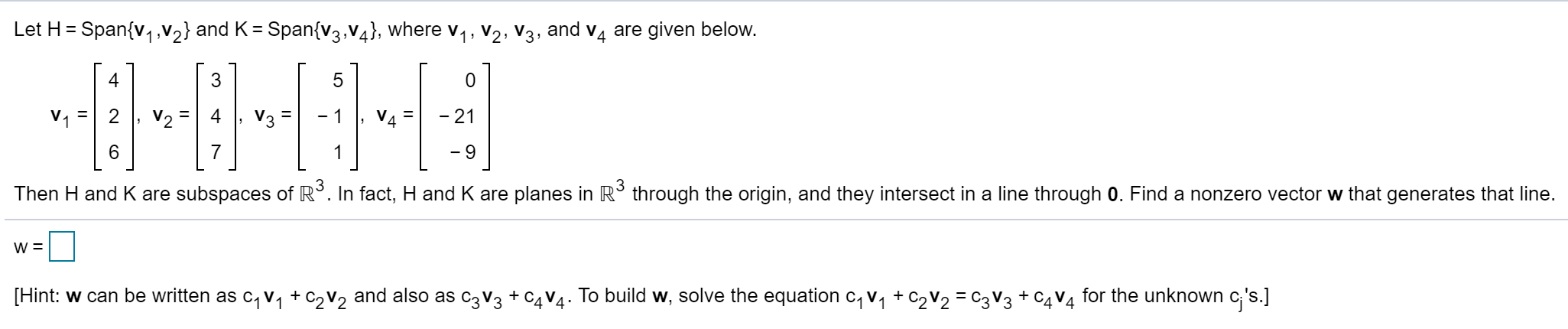Let H = Span{V1, V2} and K = Span{V3,V4}, where V1, V2, V3, and V4 are given below.   [ 0 V1 = 2 , v2 = 4, V3 = -1, V4 = -21 7 1 -9 Then H and K are subspaces of R3. In fact, H and K are planes in R3 through the origin, and they intersect in a line through 0. Find a nonzero vector w that generates that line. w=U [Hint: w can be...

• ### 3 Circuit C2 R1 Connect to Vo Unity Gair 470 0.1uF Follower Ground Output C1 4700 R2 R3 1000 C3 luF Figure 1. AC Test C...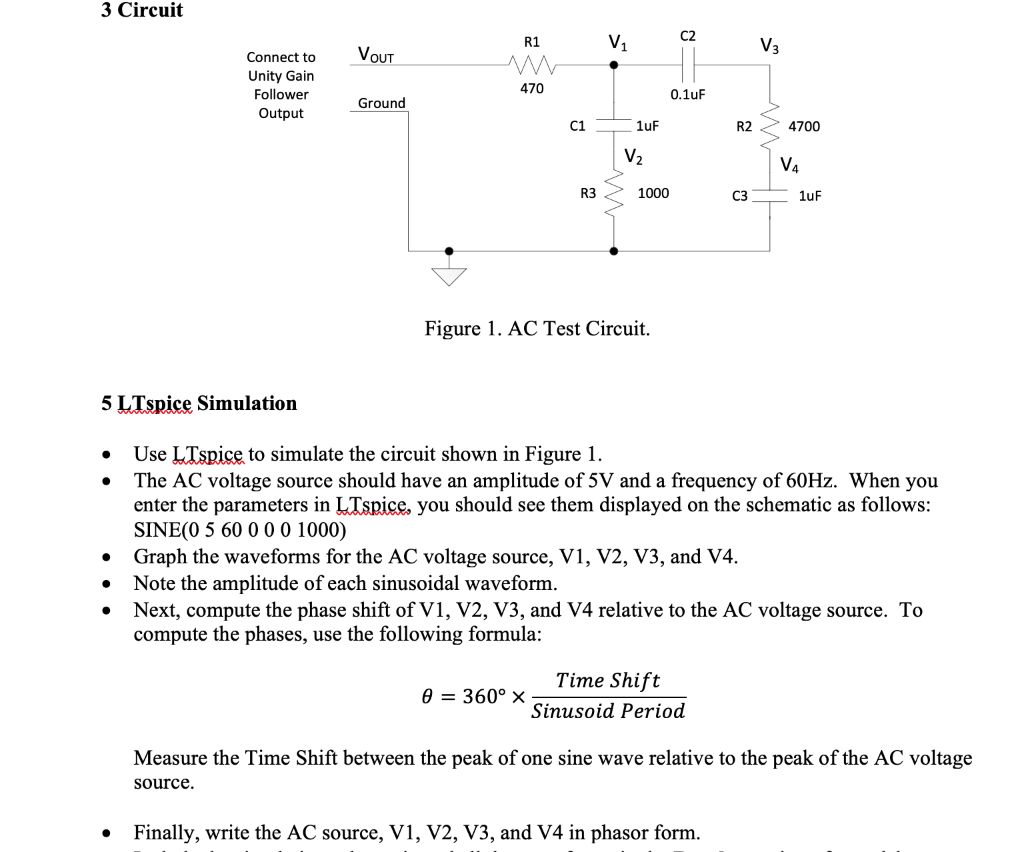3 Circuit C2 R1 Connect to Vo Unity Gair 470 0.1uF Follower Ground Output C1 4700 R2 R3 1000 C3 luF Figure 1. AC Test Circuit. 5 LTspice Simulation » Use LTspice to simulate the circuit shown in Figure 1. . The AC voltage source should have an amplitude of 5V and a frequency of 60Hz. When you enter the parameters in LTspice, you should see them displayed on the schematic as follows SINE(0 5 60000 1000) * Graph the...

• ### For the circuit shown in the figure, If R1=512, R2=10N, R3=3N, V1= V1}V, V2=5V and V3=7V....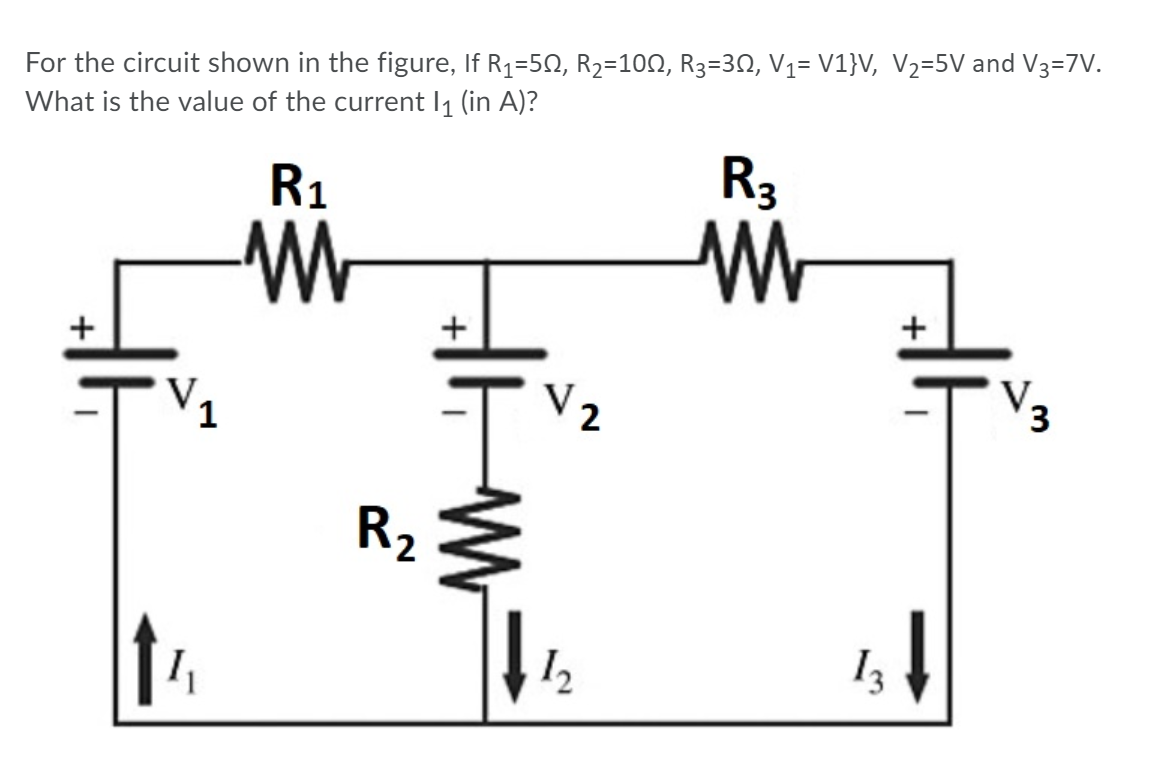For the circuit shown in the figure, If R1=512, R2=10N, R3=3N, V1= V1}V, V2=5V and V3=7V. What is the value of the current 11 (in A)? R1 -W R3 M + + V2 V. 3 R2 \$ 12 13

• ### Write the equation for loop 2 -(R6)i1 +(R6+R7+R8)13-(R8).i4=V3 0-(R5)12-(R8)i3+(R6+R7+R8)4=V2-V4 (R1+R4+R6)i 1-(R4);2-(R6) 3=V1 :-(R4)i 1+(R2+R4)2-(R5). 14-V2 QUESTION...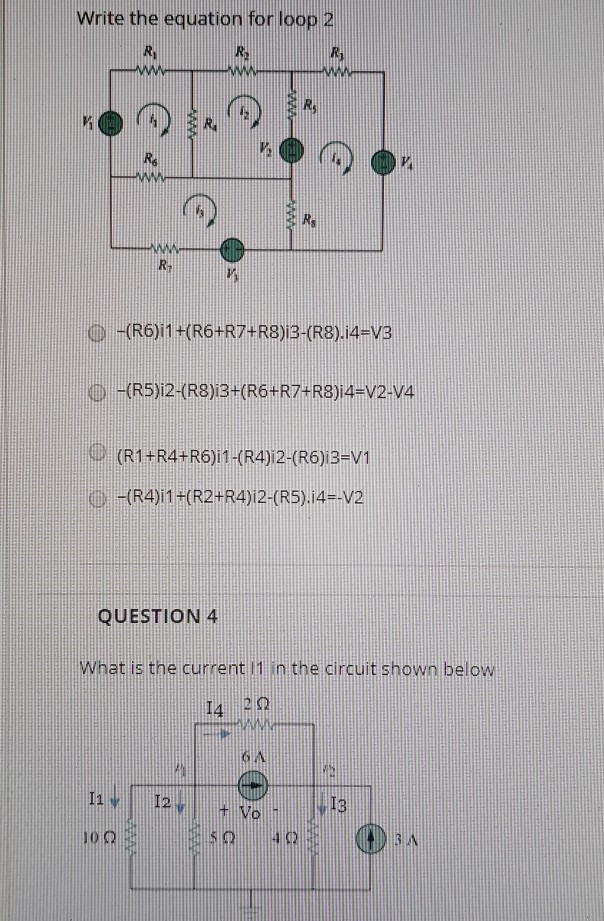Write the equation for loop 2 -(R6)i1 +(R6+R7+R8)13-(R8).i4=V3 0-(R5)12-(R8)i3+(R6+R7+R8)4=V2-V4 (R1+R4+R6)i 1-(R4);2-(R6) 3=V1 :-(R4)i 1+(R2+R4)2-(R5). 14-V2 QUESTION 4 What is the current 1 in the circuit shown below! VO SO U

• ### V1= 8V @node above R1 V2=4.64V @node between R6 and R7 V3=16.96V @node between R7 and...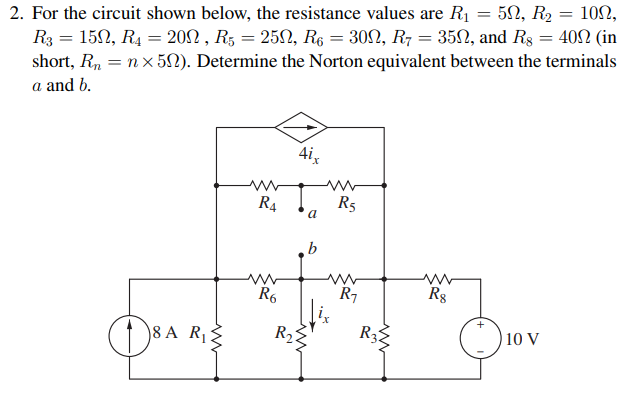V1= 8V @node above R1 V2=4.64V @node between R6 and R7 V3=16.96V @node between R7 and R8 2. For the circuit shown below, the resistance values are R1 = 512, R2 = 101, R3 = 1522, R4 = 2012 , R5 = 2522, R6 = 3032, R7 = 3512, and Rg = 4012 (in short, Rn = n x 52). Determine the Norton equivalent between the terminals a and b. Ro i 18A R} } R 10 V

• ### calculate voltage v1,v2,v3 and VS r1=1k r2=2k r3=3.9k r4=5.6k Tabla 3-1 Resultados de Divisor de Voltaje...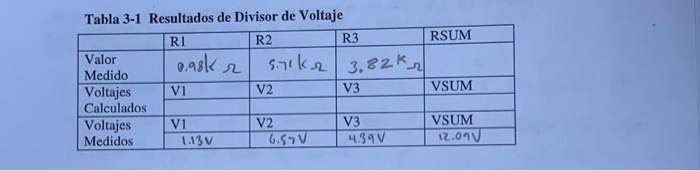calculate voltage v1,v2,v3 and VS r1=1k r2=2k r3=3.9k r4=5.6k Tabla 3-1 Resultados de Divisor de Voltaje Ri - R3 RSUM Valor 0.98k r snike 3.82k ? Medido Voltajes V1 V2 V3 VSUM Calculados Voltajes V2 VSUM Medidos I 1.130 6.57V 4.39V I 12.000 VI V3 m Vn Iv> | 12 | Ts / Id Ous SR2 3 R3 RA I 2 = Un R2 Is=Izt IstJA Vs. Un On A Un ton R1 R ik un (12V) 1926 - RIAL...

• ### V1 R7 R9 12V R3 R8 R11 R1 R2 R10 13 5A R6 R4 12 1A...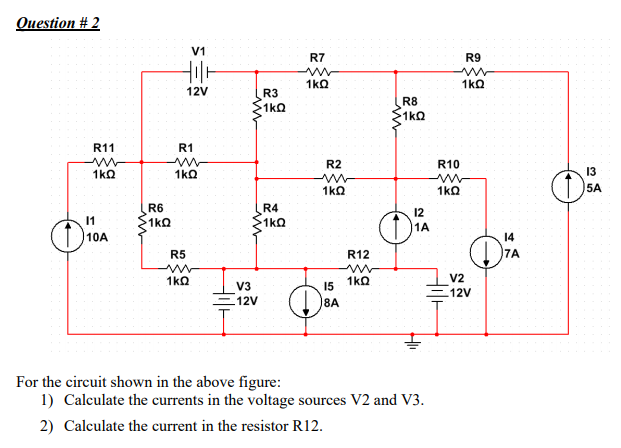V1 R7 R9 12V R3 R8 R11 R1 R2 R10 13 5A R6 R4 12 1A 10A 14 R5 R12 7A V3 12V 15 8A V2 12V For the circuit shown in the above figure 1) Calculate the currents in the voltage sources V2 and V3 2) Calculate the current in the resistor R12.

• ### To V2 - V4 R1 1 k 2 10 V For the circuit above, assuming ideal...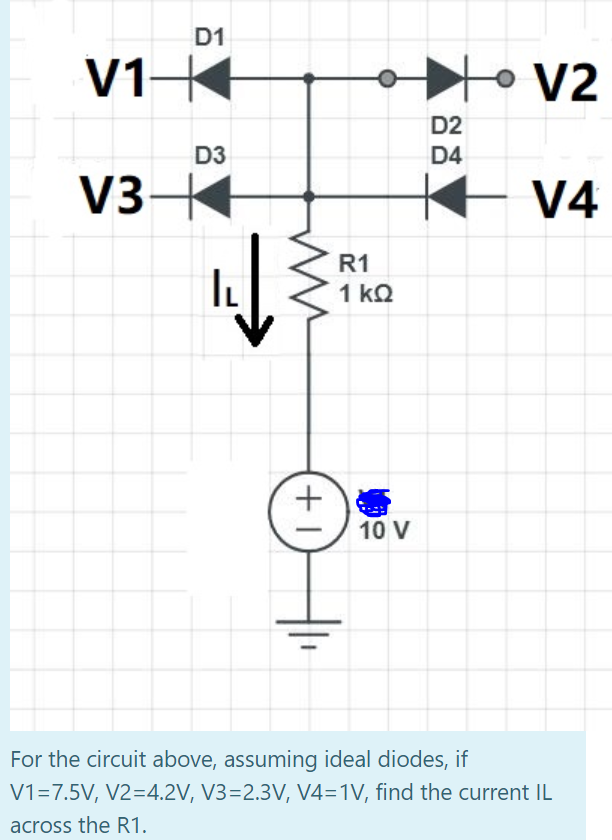To V2 - V4 R1 1 k 2 10 V For the circuit above, assuming ideal diodes, if V1=7.5V, V2=4.2V, V3=2.3V, V4=1V, find the current IL across the R1.

• ### PROTEUS +15V R5 1M R3 750 C3 HE Dvout luf Q1 ZVN2110 R1 C1 R4 100F...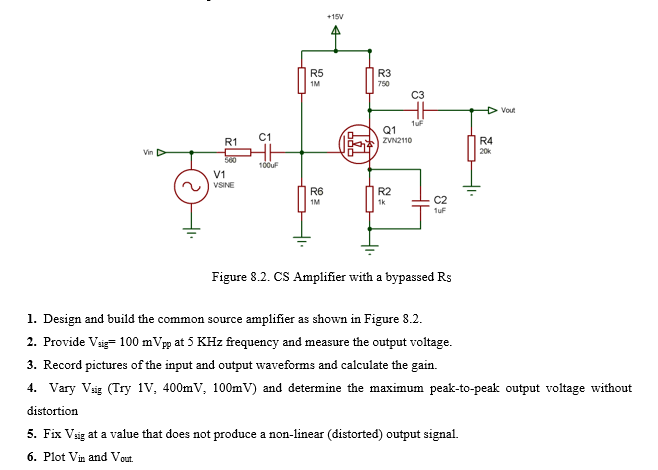PROTEUS +15V R5 1M R3 750 C3 HE Dvout luf Q1 ZVN2110 R1 C1 R4 100F V1 VSINE R6 1M R2 1k C2 Tur Figure 8.2. CS Amplifier with a bypassed Rs 1. Design and build the common source amplifier as shown in Figure 8.2. 2. Provide Vai= 100 m Vpp at 5 KHz frequency and measure the output voltage. 3. Record pictures of the input and output waveforms and calculate the gain. 4. Vary Vsig (Try 1V, 400mV, 100mV)...